# A triangular coil of wire with a height of 0.10 m, base length of 0.05 m,...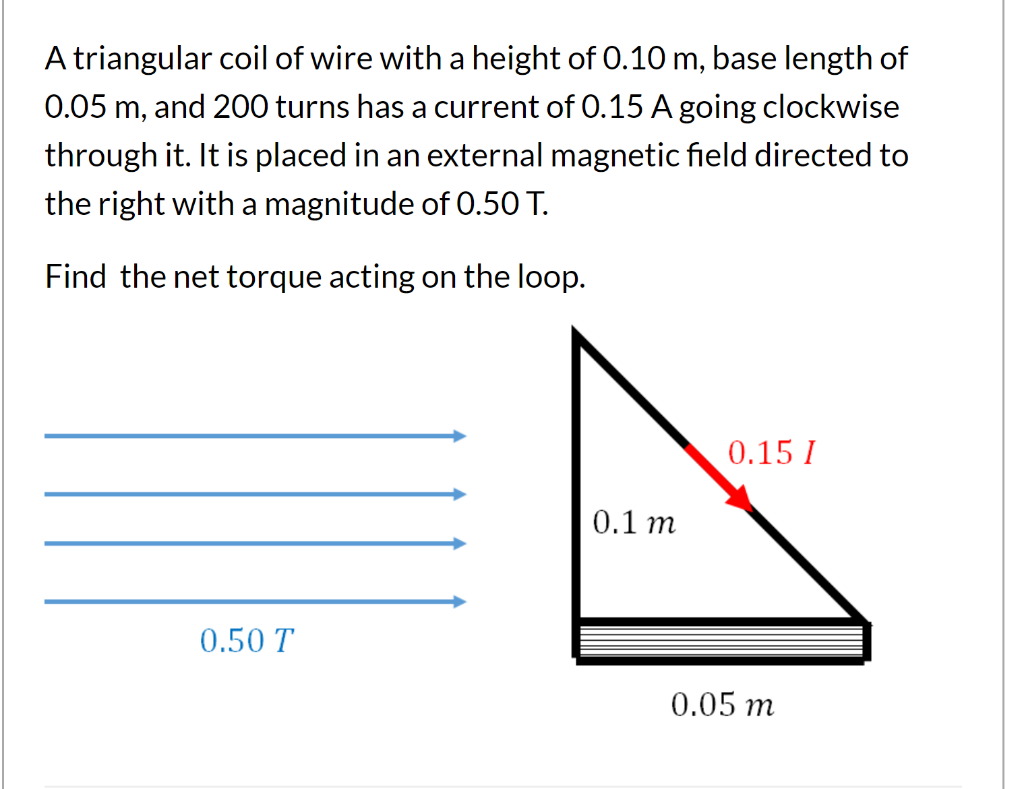A triangular coil of wire with a height of 0.10 m, base length of 0.05 m, and 200 turns has a current of 0.15 A going clockwise through it. It is placed in an external magnetic field directed to the right with a magnitude of 0.50 T. Find the net torque acting on the loop. 0.15 1 0.1 m 0.50 T 0.05 m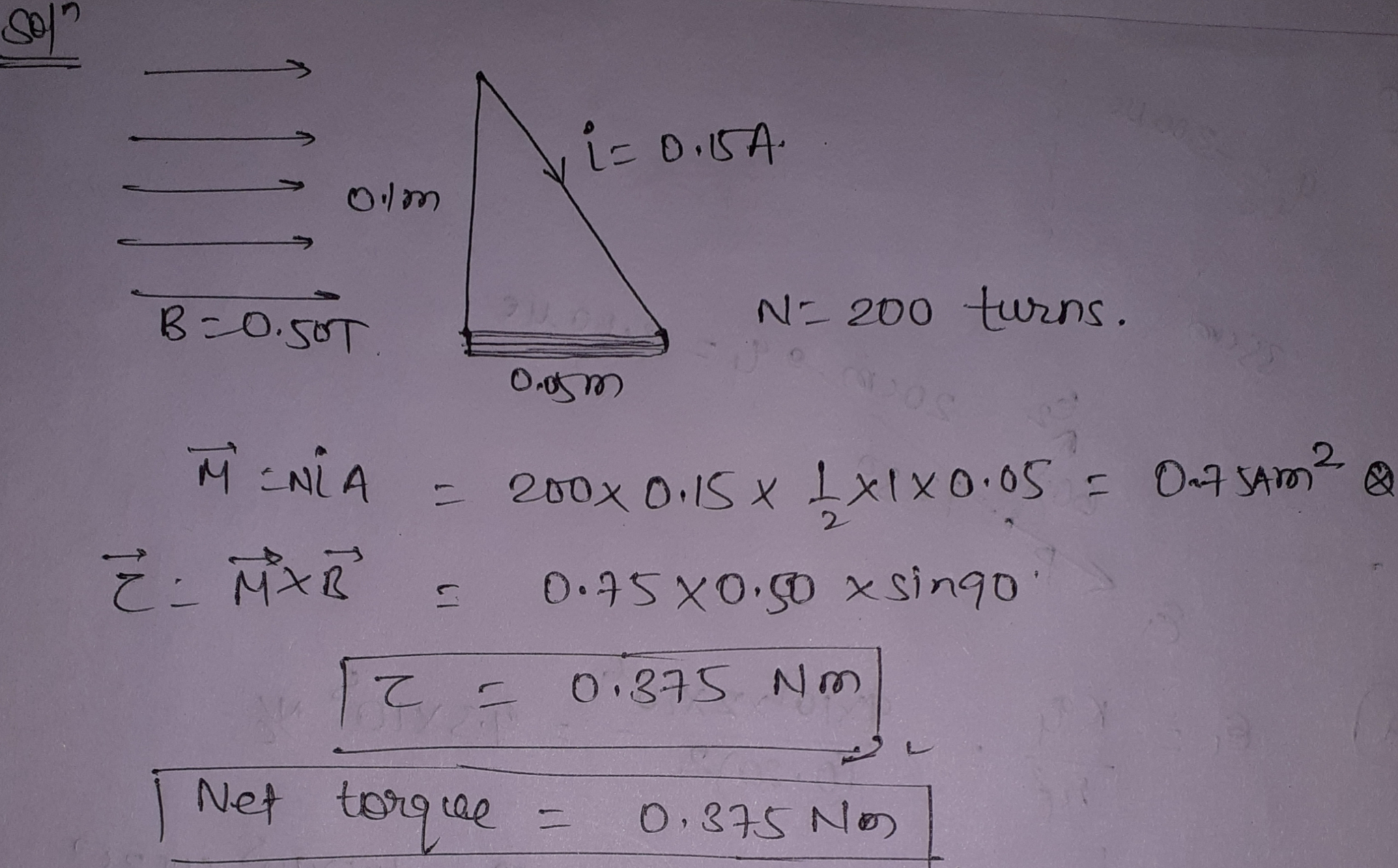##### Add Answer of: A triangular coil of wire with a height of 0.10 m, base length of 0.05 m,...
Similar Homework Help Questions
• ### An arc of wire forms a quarter circle whose radius is R relative to the origin....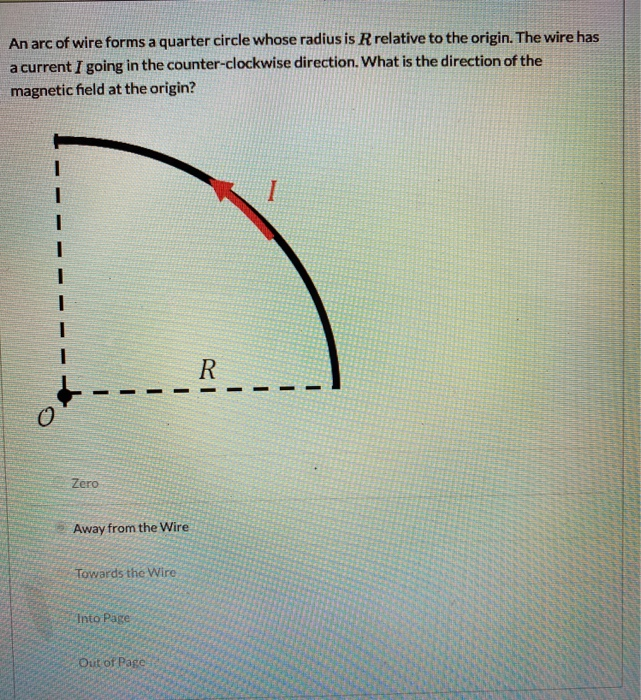An arc of wire forms a quarter circle whose radius is R relative to the origin. The wire has a current I going in the counter-clockwise direction. What is the direction of the magnetic field at the origin? I R - Zero Away from the Wire Towards the Wire Into Page Out of Page A triangular coil of wire with a height of 0.10 m, base length of 0.05 m, and 200 turns has a current of 0.15 A going...

• ### PRACTICE IT Use the worked example above to help you solve this problem. A circular wire...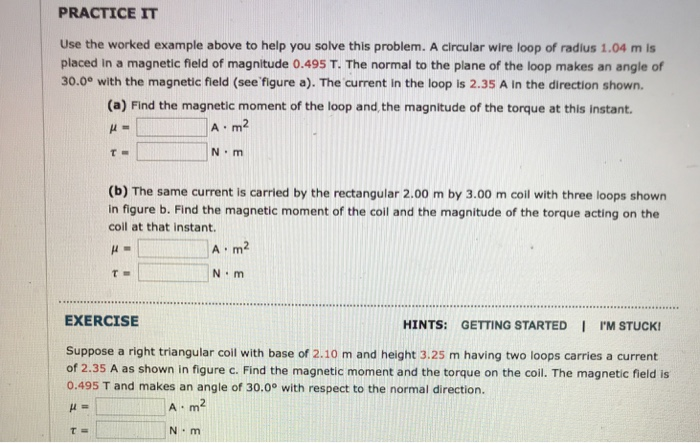PRACTICE IT Use the worked example above to help you solve this problem. A circular wire loop of radius 1.04 m is placed in a magnetic field of magnitude 0.495 T. The normal to the plane of the loop makes an angle of 30.0e with the magnetic field (see figure a). The current in the loop is 2.35 A in the direction shown. (a) Find the magnetic moment of the loop and,the magnitude of the torque at this instant. A...

• ### A rectangular coil with a length of 9.0 cm and a width of 4.0 cm and...

A rectangular coil with a length of 9.0 cm and a width of 4.0 cm and 250 turns is placed in a uniform magnetic field with a magnitude of 0.92 T. (a) If the maximum torque experienced by the coil is 0.16 N · m, determine the current in the coil. mA (b) When the magnetic field makes an angle of 35° with the normal to the plane of the coil, determine the magnitude of the torque acting on the...

• ### A rectangular current-carrying coil with sides of length a = 4.0 cm and b = 8.0 cm consists of N...

A rectangular current-carrying coil with sides of length a = 4.0 cm and b = 8.0 cm consists of N = 10closely wrapped turns, and the current through the coil is I = 0.50 A. The plane of the loop makes anangle ? = 60° with the z-axis as shown in Figure. The coil is placed in a uniform magnetic fieldTesla.a) Express the force that acts on the coil at the top of the loop.b) Express the net force acting...

• ### Chapter 21, Problem 46 GO The triangular coil of wire in the drawing is free to...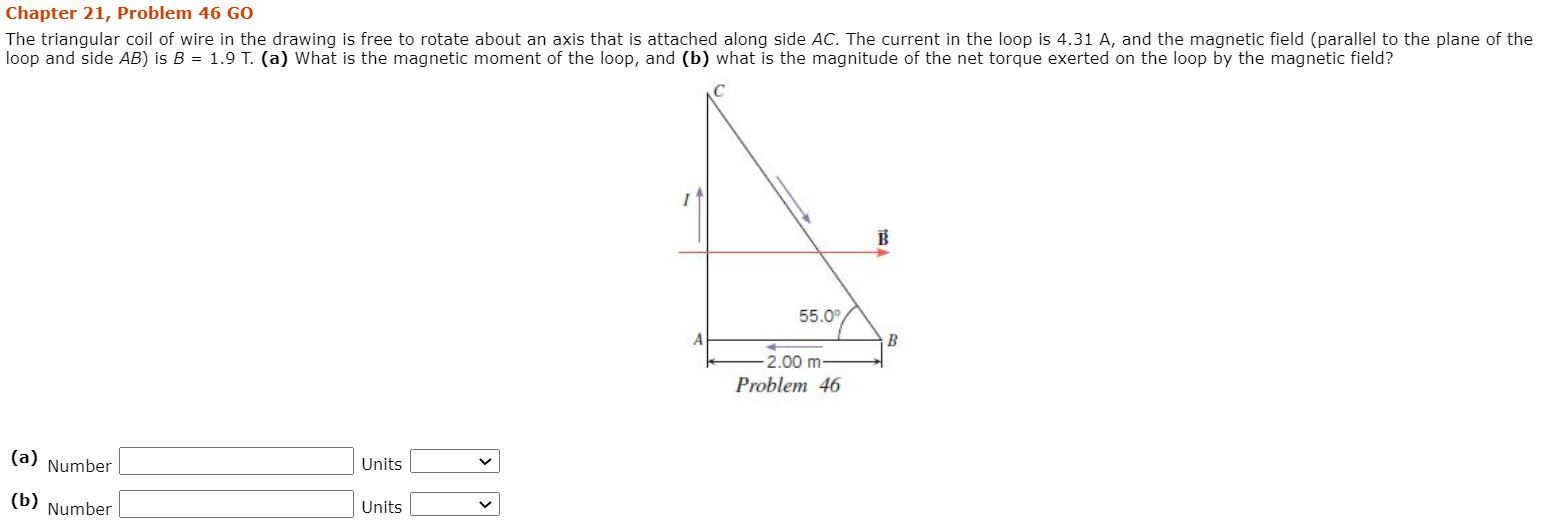Chapter 21, Problem 46 GO The triangular coil of wire in the drawing is free to rotate about an axis that is attached along side AC. The current in the loop is 4.31 A, and the magnetic field (parallel to the plane of the loop and side AB) is B = 1.9 T. (a) What is the magnetic moment of the loop, and (b) what is the magnitude of the net torque exerted on the loop by the magnetic field?...

• ### 8. A coil consists of 460 turns of wire; each having a radius of 3.80 cm....

8. A coil consists of 460 turns of wire; each having a radius of 3.80 cm. A current of 1.85 A flows through the coil. The coil is placed in an external magnetic field of 5.34 x 10 – 2 T. a. What is the magnitude of the maximum torque that can act on the coil in this magnetic field? b. What is the magnitude of the torque that acts on the coil when its magnetic field makes an angle...

• ### The Magnetic Dipole Moment of a Coil

A wire is bent into a circular coil of radius r = 4.3 cm with 23 turns clockwise, then continues and is bent into a square coil (length 2r) with 35 turnscounterclockwise. A current of 11.4 mA is running through the coil, and a 0.350 T magnetic field is applied to the plane of the coil.(a) What is the magnitude of the magnetic dipole moment of the coil?Find the magnetic moments of the circular part and the square part separately...

• ### A circular coil of wire with radius 2 cm and 60 turns is placed in a...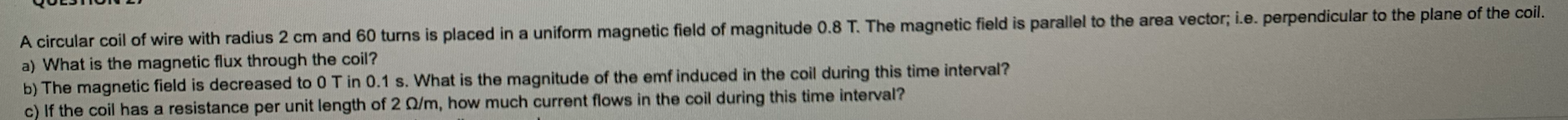A circular coil of wire with radius 2 cm and 60 turns is placed in a uniform magnetic field of magnitude 0.8 T. The magnetic field is parallel to the area vector; i.e. perpendicular to the plane of the coil. a) What is the magnetic flux through the coil? b) The magnetic field is decreased to 0 T in 0.1 s. What is the magnitude of the emf induced in the coil during this time interval? c) If the coil...

• ### will rate, thanks! A rectangular coil of wire consisting of 25.0 loops, each with length 0.200...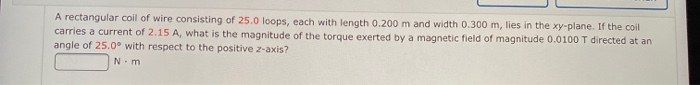will rate, thanks! A rectangular coil of wire consisting of 25.0 loops, each with length 0.200 m and width 0.300 m, lies in the xy-plane. If the coil carries a current of 2.15 A, what is the magnitude of the torque exerted by a magnetic field of magnitude 0.0100 T directed at an angle of 25.0° with respect to the positive 2-axis? N.m

• ### The 0.8 triangular wire loop above is placed in a uniform 0.4 T magnetic field directed...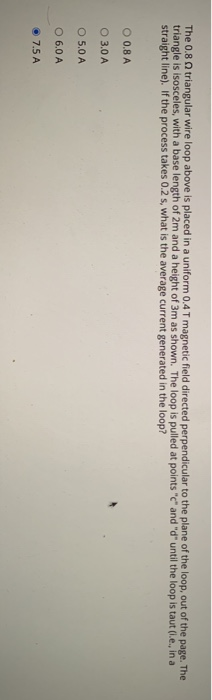The 0.8 triangular wire loop above is placed in a uniform 0.4 T magnetic field directed perpendicular to the plane of the loop, out of the page. The triangle is isosceles, with a base length of 2m and a height of 3m as shown. The loop is pulled at points "c" and "d" until the loop is taut (le, in a straight line). If the process takes 0.2 s, what is the average current generated in the loop? O 0.8...

Free Homework App